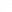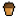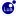# List of Jabroni Brawl: Episode 3 Script Functions's implementation of VScript is built on 's. This list only contains classes, functions and variables that differ from CS:GO's implementation.

## Constants

Instance Type Value Description
`EJBGameMode` table
```Invalid =-1
Test = 0
BattleRoyale = 1
Deathmatch = 2
FartCops = 3
GunGame = 4
Survival = 5
SvT = 6
TeamDeathmatch = 7
InstaGib = 8
Ricochet = 9
SvM = 10
HazardCourse = 11
Flagdoll = 12
ScientistHunt = 13
Hunted = 14
TeamMission = 15
RatRace = 16
HotSpot = 17
Cooperative = 18
Hidden = 19
Bonedemic = 20
GiantSlayer = 22
Max = 23
```
The gamemode of the match.
`ERoundState` table
```Invalid = -1
Init = 0
PreGame = 1
StartGame = 2
PreRound = 3
RoundRunning = 4
TeamWin = 5
Restart = 6
Stalemate = 7
GameOver = 8
Bonus = 9
BetweenRounds = 10
```
State of the round.
`EJBRoundMutator` table
```None = -1
Standard = 0
InstaGib = 1
Melee = 2
Sniper = 3
Inverted = 4
Cats = 5
HighHP = 6
Farts = 7
HighSpeed = 8
LowSpeed = 9
LowFriction = 10
FoodFight = 12
Vampirism = 14
Jousting = 15
Golden = 17
Science = 18
DuckHunt = 19
BatterUp = 20
Boomerangs = 21
BrittleBones = 22
FingerBang = 23
Ghosts = 24
GravityHell = 25
Moonwalk = 26
RedLightGreenLight = 27
Shrink = 28
Splash = 29
SlowMelon = 30
RocketPogo = 31
Mirrored = 32
Hurricane = 34
Ricochets = 35
DutyCalls = 36
TankControls = 37
Berserk = 38
ScreenSaver = 39
Max = 40
```
Mutator of the round. This only exists when Battle Royale is the current gamemode.
`ELifeState` table
```Alive = 0
Dying = 1
Respawnable = 3
```
State of an entity's lifespan.
`EJBCharacterCond` table
```None = -1
Invisible = 0
HeldUp = 2
Frozen = 3
NoDive = 4
NoWeaponDrop = 5
Dubstep = 6
SilentWalk = 8
NoThirdpersonView = 9
SilentDeath = 10
HeldUp_AllowChat = 11
GG_FinalLevel = 12
Deafen = 13
Bounce = 14
HideWeapon = 15
InvertedControls = 16
SlowWalk = 17
AutoStand = 18
SpawnProtection = 19
NoAirControl = 20
Seduced = 21
InfiniteClip = 22
InfiniteAmmo = 23
NoHealthDrop = 24
XRay = 25
AbsorbNextFall = 26
NoKicking = 27
ForceAttack1 = 28
ForceAttack2 = 29
Burning = 31
Censored = 32
Censored_Overlay = 33
Poisoned = 34
NoSlamDamage = 35
Bleeding = 36
NoFallDamage = 37
NoFireDamage = 38
InvertedControls_X = 40
InvertedControls_Y = 41
TopDownView = 42
ASCII_Overlay = 43
HPRegen = 45
CrazyViewSway = 46
HDBrown = 47
Scared = 48
Invincible = 49
Hallucinations = 50
Purgatory = 51
Predator = 52
Virus = 53
TankControls = 54
ScreenSaver = 55
NoDiveJump = 56
StompImmune = 57
Max = 58
```
Special conditions for players.
`EJBWeaponCond` table
```Golden = 0
Scoped = 1
NoPrimary = 2
NoSecondary = 3
InfiniteAmmo = 5
InfiniteClip = 6
Slowdown = 7
Max = 8
```
Special conditions for weapons.
`EJBPossessType` table
```None = -1
Pigeon = 1
Chicken = 3
Max = 4
```
Spectator monster types.

## Classes

### CBaseEntity

#### Methods

Function Signature Description
`GetLifeState` `int GetLifeState()` Returns the current life state of the entity.
`SetName` `void SetName(string name)` Sets the target name of the entity.
`SetParent` `void SetParent(handle parent)` Sets the parent of the entity.
`SetParentAttachment` `void SetParentAttachment(handle parent, int index)` Sets the parent of the entity, as well as the attachment point (by index).
`Bonemerge` `void Bonemerge(handle target)` Starts following an entity via bonemerge.

### CEntities

A client-side version of `Entities` now exists, with the following methods: `First`, `Next`, `FindByClassname`, and `GetByIndex`.

#### Methods

Function Signature Description
`Create` `handle Create(string classname, table keyvalues)` Creates and spawns an entity by classname, with the keyvalues from the given table.
`CreateNoSpawn` `handle CreateNoSpawn(string classname, table keyvalues)` Creates an entity by classname without spawning it, with the keyvalues from the given table.
`GetByIndex` `handle GetByIndex(int index)` Gets the entity at the given index.

### CBasePlayer

#### Methods

Function Signature Description
`GetUserID` `int GetUserID()` Returns player's user ID.
`GetNetworkIDString` `string GetNetworkIDString()` Returns player's Steam ID as a string.
`GetAccountID` `int GetAccountID()` Returns player's account ID.
`GetPlayerName` `string GetPlayerName()` Returns player's network name.
`IsFakeClient` `bool IsFakeClient()` Returns true if this is a fake client.

### CPlayerVoiceListener

In JBEP3, the `PlayerVoiceListener` instance has been implemented.

### CJBBaseWeapon

#### Methods

Function Signature Description
`AddCondition` `void HasCondition(int condition)` Adds the given condition to the weapon.
`HasCondition` `bool HasCondition(int condition)` Returns true if the weapon has the given condition.
`RemoveCondition` `void RemoveCondition(int condition)` Removes the given condition from the weapon.
`RemoveAllConditions` `void RemoveAllConditions()` Removes all conditions from the weapon.

### CJBPlayer

#### Methods

Function Signature Description
`AddCondition` `void AddCondition(int condition)` Adds the given condition to the player.
`HasCondition` `bool HasCondition(int condition)` Returns true if the player has the given condition.
`RemoveCondition` `void RemoveCondition(int condition)` Removes the given condition from the player.
`ResetConditions` `void ResetConditions()` Removes all conditions from the player.
`GetPossessType` `int GetPossessType()` Returns the spectator monster type of the player.

### Vector

Vectors can have each component negated with the `-` operator.

Example: `-Vector(1, -2, 3)` will evaluate to `Vector(-1, 2, -3)`

#### Methods

Function Signature Description
`constructor` `Vector Vector()` Creates a new vector (zeroed).
`constructor` `Vector Vector(float x, float y, float z)` Creates a new vector with the specified coordinates.
`operator +` `Vector Vector + Vector` Returns result of the summary.
`operator +` `Vector Vector + float` Returns a new vector with each component increased by a given number.
`operator -` `Vector Vector - Vector` Returns result of the subtraction.
`>operator -` `Vector Vector - float` Returns a new vector with each component decreased by a given number.
`operator *` `Vector Vector * Vector` Returns result of the multiplication.
`operator *` `Vector Vector * float` Returns a new vector with each component multiplied by a given number.
`operator /` `Vector Vector / Vector` Returns result of the division.

Throws an error on attempt to divide by zero.

`operator /` `Vector Vector / float` Returns a new vector with each component divided by a given number.

Throws an error on attempt to divide by zero.

`tostring` `string tostring()` Returns a human readable string.

Example output: `(vector: (0.000000, 0.000000, 0.000000))`

`ToKVString` `string ToKVString()` Returns a string that can be used when creating an entity or firing an input.

Example output: `0.000000 0.000000 0.000000`

`ToQAngle` `QAngle ToQAngle()` Returns a new QAngle.
`IsValid` `bool IsValid()` Returns true if the vector is valid, false otherwise.
`Invalidate` `void Invalidate()` Sets each component to `NaN`.
`IsZero` `bool IsZero()` Returns 'true' if the vector has zero length, 'false' otherwise.
`Zero` `void Zero()` Sets each components to zero.
`Random` `void Random(float min, float max)` Randomizes each component's value within the specified range.
`Scale` `Vector Scale(float mult)` Returns a new vector with each component multiplied by a given number.
`Lerp` `Vector Lerp(Vector other, float frac)` Returns vector interpolated a given amount between itself and another given vector.
Length float Length() Returns length of the vector.
`LengthSqr` `float LengthSqr()` Returns the squared length of the vector.
`Length2D` `float Length2D()` Returns the length of the vector, only considering the X and Y axes.
`Length2DSqr` `float Length2DSqr()` Returns the squared length of the vector, only considering the X and Y axes.
`LargestComponent` `int LargestComponent()` Returns index of the largest component.
`LargestComponentValue` `float LargestComponentValue()` Returns value of the largest component.
`SmallestComponent` `int SmallestComponent()` Returns index of the smallest component.
`SmallestComponentValue` `float SmallestComponentValue()` Returns value of the smallest component.
`Min` `Vector Min(Vector other)` Returns the minimum between this and a given vector.
`Max<` `Vector Max(Vector other)` Returns the maximum between this and a given vector.
`Normalize` `float Normalize()` Normalizes the vector, and returns the vector length.
`DistTo` `float DistTo(Vector other)` Returns the distance between the vector and a given vector.
`DistToSqr` `float DistToSqr(Vector other)` Returns the squared distance between the vector and a given vector.
`Dot` `float Dot(Vector other)` Returns the dot product of the vector and a given vector.
`Cross` `Vector Cross(Vector other)` Returns the cross product of the vector and a given vector.
`ProjectOnto` `>Vector ProjectOnto(Vector other)` Returns the vector projected onto a given vector.
`Rotate` `Vector Rotate(QAngle angles)` Returns the vector rotated by a given QAngle.

#### Members

Member Type Description
`x` `float` Cartesian X axis.
`y` `float` Cartesian Y axis.
`z` `float` Cartesian Z axis.

### QAngle

#### Methods

Function Signature Description
`constructor` `QAngle QAngle()` Creates a new QAngle (zeroed).
`constructor` `QAngle QAngle(float pitch, float yaw, float roll)` Creates a new QAngle with the specified coordinates.
`operator +` `QAngle QAngle + QAngle` Returns result of the summary.
`operator +` `QAngle QAngle + float` Returns a new QAngle with each component increased by a given number.
`operator -` `QAngle QAngle - QAngle` Returns result of the subtraction.
`operator -` `QAngle QAngle - float` Returns a new QAngle with each component decreased by a given number.
`operator *` `QAngle QAngle * QAngle` Returns result of the multiplication.
`operator *` `QAngle QAngle * float` Returns a new QAngle with each component multiplied by a given number.
`operator /` `QAngle QAngle / QAngle` Returns result of the division.

Throws an error on attempt to divide by zero.

`operator /` `QAngle QAngle / float` Returns a new QAngle with each component diivided by a given number.

Throws an error on attempt to divide by zero.

`tostring` `string tostring()` Returns a human readable string.

Example output: `(qangle: (0.000000, 0.000000, 0.000000))`

`ToKVString` `string ToKVString()` Returns a string that can be used when creating an entity or firing an input.

Example output: `0.000000 0.000000 0.000000`

`IsValid` `boolean IsValid()` Returns true if the QAngle is valid, false otherwise.
`Invalidate` `void Invalidate()` Sets each component to `NaN`.
`Random` `void Random(float min, float max)` Randomizes each component's value within the specified range.
`Scale` `QAngle Scale(float mult)` Returns a new QAngle with each component multiplied by a given number.
`Length` `float Length()` Returns the length of the QAngle.
`LengthSqr` `float LengthSqr()` Returns the squared length of the QAngle.
`Normalize` `void Normalize()` Clamps each component in range (-180..180]
`NormalizePositive` `void NormalizePositive()` Clamps each component in range [0..360)
`Forward` `Vector Forward()` Returns a normal vector in the direction that the QAngle points.
`Right` `Vector Right()` Returns a normal vector pointing to the right of the direction that the QAngle points.
`Up` `Vector Up()` Returns a normal vector pointing upwards of the direction that the QAngle points.

#### Members

Member Type Description
`x` `float` Rotation on the X axis.
`y` `float` Rotation on the Y axis.
`z` `float` Rotation on the Z axis.
`pitch` `float` Same as `x`.
`yaw` `float` Same as `y`.
`roll` `float` Same as `z`.

## Global classes

### g_Clients

#### Methods

Function Signature Description
`GetLocalPlayer` `handle GetLocalPlayer()` Client-side only. Gets the local player.
`IsClientConnected` `bool IsClientConnected(int index)` Server-side only. Returns true if the client at the given index is connected.
`IsClientInGame` `bool IsClientConnected(int index)` Server-side only. Returns true if the client at the given index is in-game.
`IsFakeClient` `bool IsFakeClient(int index)` Server-side only. Returns true if the client at the given index is fake.
`IsClientSourceTV` `bool IsClientSourceTV(int index)` Server-side only. Returns true if the client at the given index is SourceTV.
`GetMaxClients` `int GetMaxClients()` Returns the maximum number of clients.
`GetPlayerByIndex` `handle GetPlayerByIndex(int index)` Gets the player at the given index. Index starts at 1.
`GetPlayerByUserID` `handle GetPlayerByUserID(int userid)` Gets a player from the given user ID.
`GetPlayerBySteamID` `handle GetPlayerBySteamID(string steamid)` Gets a player from the given Steam ID. Uses the `STEAM_X:Y:Z` format.
`GetPlayerByAccountID` `handle GetPlayerByAccountID(int accountid)` Gets a player from the given Steam account ID.

### g_CVar

#### Methods

Function Signature Description
`GetFloat` `float GetFloat(string convar)` Returns the given convar as a float.
`GetString` `string GetString(string convar)` Returns the given convar as a string.
`GetClientConVarValue` `string GetClientConVarValue(string convar, int index)` Returns the given convar for the entity at the given index as a string.
`SetValue` `void SetValue(string convar, variant value)` Sets the value of the given convar to the given value. Supported types are `bool`, `int`, `float`, `string`.

### g_GameRules

#### Methods

Function Signature Description
`IsMultiplayer` `bool IsMultiplayer()` Returns true if the current gamemode is multiplayer.
`IsTeamplay` `bool IsTeamplay()` Returns true if the current gamemode is team-based.
`IsCoOp` `bool IsCoOp()` Returns true if the current gamemode is cooperative.
`GetRoundState` `int GetRoundState()` Returns the state of the current round.
`IsInWaitingForPlayers` `bool IsInWaitingForPlayers()` Returns true if the game is currently waiting for players before starting.
`GetModeID` `int GetModeID()` Returns the ID of the current gamemode.
`GetModeName` `string GetModeName()` Returns the short name of the current gamemode.
`GetModeNameLong` `string GetModeNameLong()` Returns the long name of the current gamemode.
`GetRoundMutator` `int GetRoundMutator()` Returns id of the current round's mutator.

## Global functions

### Printing and drawing

Function Signature Description
`__DevMsg` `void __DevMsg(int developerLevel, string message)` Prints the given message to the developer console if the current developer level is equal to or greater than the given developer level.
`DevMsg` `void DevMsg(string message)` Prints the given message to the developer console if the current developer level is equal to or greater than 1.
`DevMsg2` `void DevMsg2(string message)` Prints the given message to the developer console if the current developer level is equal to or greater than 2.
`DevMsg3` `void DevMsg3(string message)` Prints the given message to the developer console if the current developer level is equal to or greater than 3.
`DebugDrawText` `void DebugDrawText(Vector origin, string text, bool bViewCheck, float duration)` Draw text in 3D, at the given origin.
`DebugDrawScreenTextLine` `void DebugDrawScreenTextLine(float x, float y, int lineOffset, string text, int r, int g, int b, int a, float duration)` Draw text in 2D, at the given X and Y coordinates, with a line offset.
`DebugDrawLine_vCol` `void DebugDrawLine_vCol(Vector start, Vector end, Vector rgb, bool zTest, float duration)` Draw a debug line between the given positions.
`DebugDrawCircle` `void DebugDrawCircle(Vector center, float radius, Vector rgb, bool zTest, float duration)` Draw a debug circle, at the given origin.
`DebugDrawBoxDirection` `void DebugDrawBoxDirection(Vector center, Vector min, Vector max, Vector forward, Vector rgb, float alpha, float duration)` Draw a debug forward box.
`DebugDrawClear` `void DebugDrawBoxDirection()` Try to clear all the debug overlay info.

### Other

Function Signature Description
`IsServerRealm` `bool IsServerRealm()` Returns true if the script is running on a server.
`IsDedicatedServer` `bool IsDedicatedServer()` Returns true if the script is running on a dedicated server.
`DispatchSpawn` `void DispatchSpawn(handle entity)` Spawns an entity that has been created but not spawned yet.

### Removed functions

Function Signature Description Reason
`CreateProp` `handle CreateProp(string classname, Vector origin, string model, int animation)` Create a prop with the specified class and model. Both prop_physics, prop_dynamic as well as some other entity classes with models work. Does not precache the model. Broken, and no longer needed with the addition of `Create` and `CreateNoSpawn`.
`GetDeveloperLevel` `int GetDeveloperLevel()` Gets the level of the developer mode. Equivalent to `developer()`. Removed in favor of `developer()`.

## Global hooks

Function Signature Description
`OnGameEvent` `void OnGameEvent(string eventName, table eventData)` Called whenever an event is triggered. Can be used to control various events with varying conditions. eventData will hold the elements for various conditions of the event.